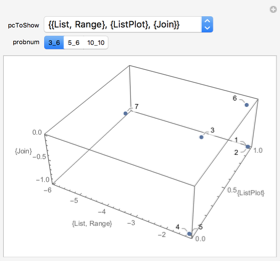#Wolfram Summer School

# Alumni# Lauren Berk

Educational Innovation

Class of 2018

## Bio

Lauren Berk is a fifth-year PhD student in operations research at MIT, working with Prof. Robert Freund and Prof. Craig Carter. Her dissertation research introduces new techniques for data analysis and optimization in educational technology. She is working with others at the Summer School to use the Wolfram Language to teach physics and computing to undergraduates at MIT. Before starting her PhD, Lauren earned her bachelor’s degree in mathematics from Yale University and worked as a consultant at an operations research and business analytics firm, Analytics Operations Engineering.

## Computational Essay

Visualizing the Bolzano–Weierstrass Theorem »

## Project: Clustering and Visualizing Submitted Solutions to An Elementary Introduction to the Wolfram Language

### Goal

To create a function analogous to Classify that takes in a programming problem and student solutions (some correct, some incorrect) and builds a classifier that can determine if a given program is correct or incorrect and, more specifically, why the solution is correct or incorrect. (If correct, which approach was taken. If incorrect, what was done in error or misunderstood?) We will develop this function using data from the An Elementary Introduction to the Wolfram Language problems and Wolfram Challenges.

### Main Results in Detail

We created a function that takes in student solutions to arbitrary An Elementary Introduction to the Wolfram Language problems, collects the key components of the solutions and clusters the solutions in order to understand common approaches to solving (or failing to solve) each problem. We performed a truncated principal component analysis on the results so we could visualize the locations of these clusters in the space of symbol use.

### Future Work

We intend to go much deeper into analyzing this data and data from Wolfram Challenges. We will explore the graph representation of code to generate additional distance metrics for clustering, look at the performance of users over time and over problems and generate automated helpful suggestions.APPLY NOW